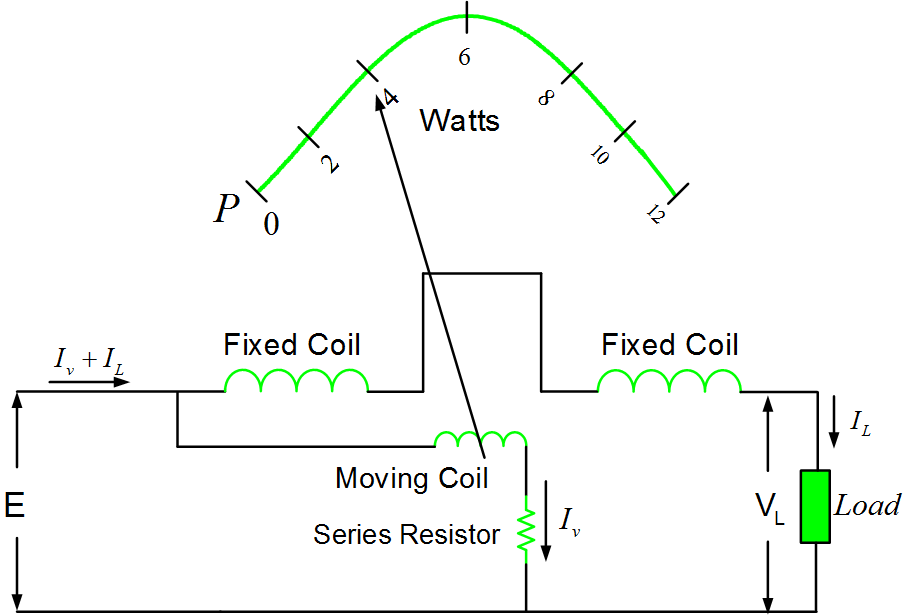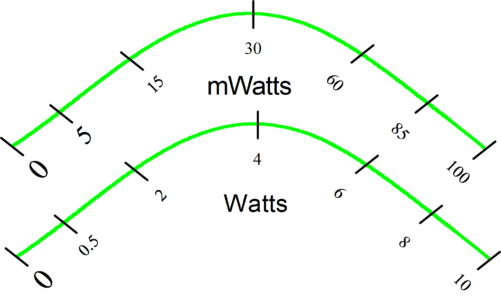Home / Instrumentation / Dynamometer Type Wattmeter Working Principle | Electrodynamometer

# Dynamometer Type Wattmeter Working Principle | Electrodynamometer

Want create site? Find Free WordPress Themes and plugins.

In a dc circuit, the power supplied to a load can be determined by measuring the load voltage and current and multiplying them together:

P=EI

However, it is much more convenient to have an instrument that indicates power directly. The meter used for this purpose is called a wattmeter, and the instrument that can be applied as a wattmeter is known as a dynamometer, or sometimes as an electrodynamic instrument. The construction of a dynamometer instrument to some extent resembles the PMMC instrument. The major difference from the PMMC construction is that the permanent magnet is replaced by two coils, as illustrated in figure (1). The magnetic field in which the lightweight moving coil is situated is generated by passing current through the fixed field coils. Then, when a current is passed through the moving coil, the moving coil and the meter pointer are deflected.Fig.1: Dynamometer Instrument

The deflection of the pointer of a dynamometer instrument is proportional to the current through the moving coil, but it is also proportional to the flux density of the magnetic field set up by the fixed field coils. This means, of course, the deflection is also proportional the current through the fixed field coils.

Consider the arrangement shown in figure 1. The moving coil of the instrument has a series resistor and is connected in parallel with the load. Consequently, current Iv through the moving coil is directly proportional to the load voltage. The field coils are connected in series with the load, so the current flowing through them is (Iv+IL), as shown. If Iv<<IL, Iv can be neglected and the field coil current assumed to be approximately equal to IL. Because the meter deflection is proportional to the field coil current and to the moving coil current,

$deflection\text{ }\alpha \text{ }V{}_{L}*{{I}_{L}}$

or

$deflection\text{ }\alpha \text{ }P$

The scale of the instrument can be calibrated to indicate watts, and thus it becomes a dynamometer type wattmeter.

The scale of the wattmeter is illustrated in figure 2 in which upper scale is calibrated in milli-watts while the lower one measures the power in watts range.Fig.2: Wattmeter Scale

Because fairly large currents are required to set up the necessary field flux, the dynamometer instrument is not as sensitive as a PMMC instrument. Consequently, its major application is as a wattmeter. One advantage that the dynamometer has over a PMMC instrument is that it can be used for both direct and alternating current/voltage instruments.

Did you find apk for android? You can find new Free Android Games and apps.

### About Ahmad FaizanMr. Ahmed Faizan Sheikh, M.Sc. (USA), Research Fellow (USA), a member of IEEE & CIGRE, is a Fulbright Alumnus and earned his Master’s Degree in Electrical and Power Engineering from Kansas State University, USA.